# Machine learning models behind targeted display advertising. Explained.

You might have heard of the famous phrase “If you are not paying for the product then, You are not the Customer, YOU are the product”. This cannot be more accurate in the age of free access to social media. You may have just searched for an item on your favorite shopping website and all of a sudden see recommendations related to your search haunt the rest of your browsing session or beyond. This is just one such example where targeted ads are used by companies for generating revenue. More sinister use cases include the infamous Cambridge analytica scandal which was the birth child of the concept of targeted advertising.

In this article we will analyze which machine learning models can be used for targeted ads, implement the models in a real world scenario and compare the accuracy of the results between the models. The two ML models under consideration are the ‘Logistic regression’ and the ‘K-nearest neighbours (KNN)’ models.

Logistic Regression model

Consider a scenario where a plot containing data points of whether a person would perform a particular action with relation to their age (Let’s say a person is of age X and has clicked a particular link or not). Here 0 indicates the person has not performed a particular action where as a 1 indicates that the person in question has indeed performed the action (see fig). When we proceed to draw a regression plot along the data points, to an extent it does establish a trend (In this case, a person of a higher age is more likely to perform the action compared to a younger person). However, the linear regression plot doesn’t look like it’s best suited for prediction because of the outliers above 1 and beyond 0.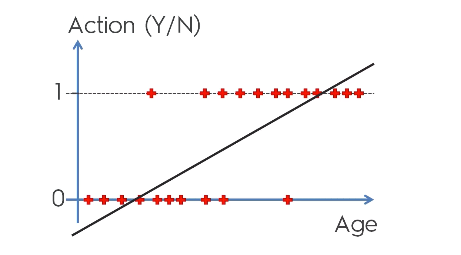Linear regression plot

We would rather want to take an probabilistic approach which indicates the likelihood of whether a person would perform the action or not and for that the prediction has to lie between 0 and 1. In order to convert the linear plot to fit between 0 and 1, we can apply a mathematical function called a ‘Sigmoid function’.

Once the sigmoid function is applied, we get a curve of best fit which covers all our data points and the model is called the ‘Logistic regression model’. We can now go on to say that for a particular age if the corresponding probability is greater than a certain value (let’s say 0.5 or 50%) then they are more likely to perform that particular action.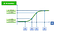Logistic regression model

When the age in the X axis is projected to the curve, we get the ‘fitted values’ (indicated in blue cross). When the fitted values are projected to the Y axis, it gives us the probability value for the corresponding age in the X axis.

Building a Logistic regression model in Python

Let’s consider a case where we have a data set of users of certain age, gender, estimated salary and whether they own a SUV vehicle or not. Our task is to use the logistic regression model to see how accurately does it predict the result and then compare it with the data set which we are using.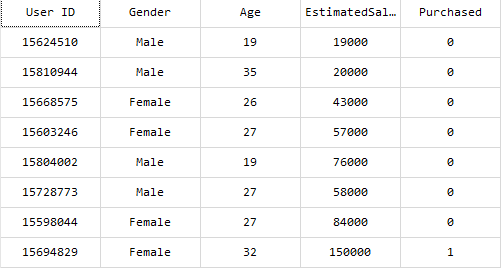Sample of the data set used for predicting if a user has purchased an SUV

To create a logistic regression model in Python, the Scikit-learn API is used. Once the required libraries and data set is imported, the data is the split into test and training set meaning a portion (let’s say 75%) is used for training the model and the rest is used for testing how accurate the predictions are. It’s also important that all the dependent variables are in the same range of values and hence ‘feature scaling’ is performed as a part of the data pre- processing. Once the model is trained based on the training data set, the test and the training results are predicted. In order to gauge the accuracy of the prediction in the training and test sets, a 2x2 prediction matrix called the ‘confusion matrix’ is created.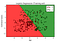Prediction result of training data set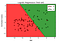Prediction result of test data set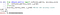Confusion matrix of the prediction results

Interpreting the prediction results

As seen the above results, the prediction boundary is a straight line because the logistic regression model is a ‘linear classifier’. The region in red indicates that the person has not purchased a SUV and the region in green indicates the person has made the purchase. The same color code holds for the data points which are distributed in the two regions. As seen in the prediction results, there are a few red data points in the green region and vice versa indicating an error in prediction by the model. The confusion matrix gives a count of how many predictions were correct and how many of them were inaccurate based on our data set. According to the confusion matrix (see fig above) in the training set, 65 predictions were accurate and 8 were inaccurate. Likewise in the test set 24 predictions were found to be accurate and 3 were inaccurate resulting in an overall accuracy of 89%. Since logistic regression uses a straight line to separate the regions (linear classifier) a few data points may end up on the wrong side of the prediction. Can that be avoided if the separator was something other than a straight line? (Let’s say a curve). We examine that in the next section.

K-Nearest neighbors (KNN) model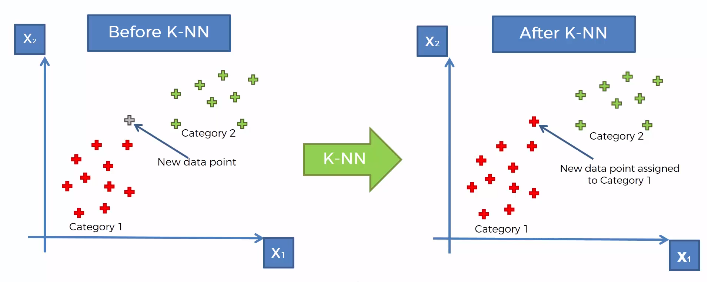K-Nearest neighbors classification

When a new data point is added, the big question is how do you categorise the data point. How exactly do you predict which category the data point should belong to? The K-Nearest neighbors classification model answers that question. In the context of our problem, it can predict whether the user is likely to purchase the SUV or not. Before we proceed with the steps to implement the KNN model it is important to be clear with the simple mathematical concept of ‘Euclidean distance’.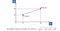Euclidean distance between two points

The Euclidean distance gives us the distance between the two points based on their co-ordinates. However, there is no steadfast rule that says that only euclidean distance has to be used to measure the distance between the data points. Other methods such as the Manhattan distance can also be used.

Building a KNN model in Python

As a first step in building a KNN model, we choose the number of neighbours to be considered (let’s say K=5). The next step is to find the euclidean distance between the new data point and the five nearest data points. Next, count the number of data points in each category (see fig below).

As seen above, the category 1 has 3 neighbouring data points as opposed to 2 neighbouring data points in category 2. Hence we assign the new data point to category 1. Simple right!? To build a KNN model in Python Scikit learn API can be used.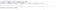Building a KNN model in Python using Scikit learn API

Interpreting the prediction results

As seen the above results, the prediction boundary is a curved line because the KNN model is a ‘non linear classifier’. The region in red indicates that the person has not purchased a SUV and the region in green indicates the person has made the purchase. The same color code holds for the data points which are distributed in the two regions. As seen in the prediction results, there are very few red data points in the green region and vice versa indicating an highly accurate prediction by the model. The confusion matrix gives a count of how many predictions were correct and how many of them were inaccurate based on our data set. According to the confusion matrix (see fig below) in the training set, 64 predictions were accurate and only 3 were inaccurate. Likewise in the test set 29 predictions were found to be accurate and 4 were inaccurate resulting in an overall accuracy of above 90% (which indicates an improvement in performance compared to the logistic regression model). Since KNN uses a curved line to separate the regions (non linear classifier) very few data points may end up on the wrong side of the prediction.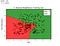Confusion matrix and training set prediction results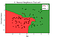Test set prediction result

Conclusion

Both Logistic regression (linear classifier)and the K nearest neighbors (non linear classifier) are excellent ML models which can be used for target ads. While KNN model showed an improvement in accuracy in prediction of about 4%, on the downside of KNN is that it’s more compute intensive compared to the logistic regression model. These trade offs have to be considered while using the right model for the targeted ad application.

The source code for this project can be found here https://github.com/mukul-keerthi

The author works as an embedded software engineer in the lovely mid-west of Ireland!

## More from Mukul Keerthi

The author works as an embedded software engineer in the lovely mid-west of Ireland!